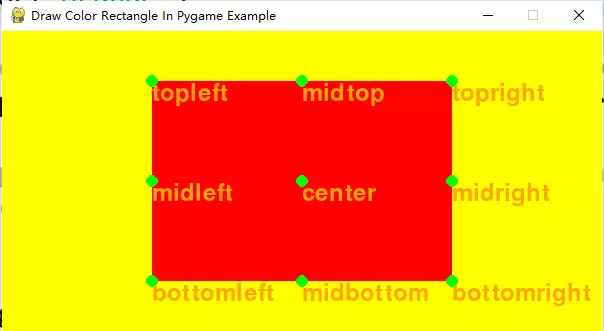# How To Draw A Color Rectangle In Pygame

The python pygame.Rect(top, left, width, height) class is used to create a rectangle object. You can specify the rectangle object’s position (top, left) and size (width, height) when you create it. And the rectangle represented area must be drawn on a pygame surface object. This article will tell you how to use the pygame.Rect object to draw a rectangle with a provided color.

### 1. How To Draw A Color Rectangle In Pygame Steps.

1. First, create an instance of the pygame.Rect class and provide the top, left point coordinates and the rectangle object width and height.
`rect_object = pygame.Rect(rect_left_top_point_x, rect_left_top_point_y, rect_width, rect_height)`
2. Then you can use the method pygame.draw.rect() to draw the rectangle object on the surface object.
`pygame.draw.rect(main_window_screen, rect_color, rect_object)`
3. Below is the python source code of this example, you can see the pygame.Rect object has a lot of attributes ( top, left, topleft, bottomright, center, midleft, midright .etc) to get the coordinates of the different points on the rectangle object.
```'''
Created on Feb 23, 2022

@author: Jerry Zhao
'''

import pygame, sys

# define the pygame main window size.
MAIN_WINDOW_SIZE = (600,300)

# define a tuple to save all the pygame.Rect key points coordinate's attribute names.
RECT_KEY_POINTS_ATTR_NAMES_TUPLE = ('topleft', 'topright', 'bottomleft', 'bottomright', 'midtop', 'midright', 'midbottom', 'midleft', 'center')

# this function will draw a rectangle with provided color.
def draw_a_color_rectangle_example():

# initialize pygame application.
pygame.init()

# create the pygame application main window surface object.
main_window_screen = pygame.display.set_mode(MAIN_WINDOW_SIZE)

# set the main window title.
pygame.display.set_caption('Draw Color Rectangle In Pygame Example')

# define the rectangle object top, left coordinates values.
rect_left_top_point_x = 150
rect_left_top_point_y = 50

# get the main window width and height.
main_window_width = main_window_screen.get_width()
main_window_height = main_window_screen.get_height()

# calculate the rectangle object's width and height.
rect_width = main_window_width - 2 * rect_left_top_point_x
rect_height = main_window_height - 2 * rect_left_top_point_y

# create the pygame.Rect object use the above parameters.
rect_object = pygame.Rect(rect_left_top_point_x, rect_left_top_point_y, rect_width, rect_height)

print('main_window_width = ', main_window_width)

print('main_window_height = ', main_window_height)

print('rect_object.x = ', rect_object.x)

print('rect_object.y = ', rect_object.y)

print('rect_object.width = ', rect_object.width)

print('rect_object.height = ', rect_object.height)

print('rect_object.left = ', rect_object.left)

print('rect_object.top = ', rect_object.top)

print('rect_object.right = ', rect_object.right)

print('rect_object.bottom = ', rect_object.bottom)

print('rect_object.center = ', rect_object.center)

print('rect_object.topleft = ', rect_object.topleft)

print('rect_object.topright = ', rect_object.topright)

print('rect_object.bottomleft = ', rect_object.bottomleft)

print('rect_object.bottomright = ', rect_object.bottomright)

print('rect_object.midleft = ', rect_object.midleft)

print('rect_object.midright = ', rect_object.midright)

print('rect_object.midtop = ', rect_object.midtop)

print('rect_object.midbottom = ', rect_object.midbottom)

# create a pygame.font.Font object with the provided font size.
font = pygame.font.Font(None, 36)

# the pygame application's main loop.
while True:

for event in pygame.event.get():

if event.type == pygame.QUIT:

pygame.quit()

sys.exit(0)

# set the main window screen background color.
main_window_background_color = pygame.Color('yellow')
main_window_screen.fill(main_window_background_color)

# set the rectangle color.
rect_color = pygame.Color('red')
pygame.draw.rect(main_window_screen, rect_color, rect_object)

# loop all the pygame.Rect key points attribute names.
for key_point_attribute_name in RECT_KEY_POINTS_ATTR_NAMES_TUPLE:

# get the pygame_rectangle_object.key_point_attribute representation.
point_position = eval('rect_object.' + key_point_attribute_name)

# define the text color.
text_color = pygame.Color('orange')

# create the text surface object with the provided text(key_point_attribute_name) and color.
text = font.render(key_point_attribute_name, True, text_color)

# draw the text on the pygame application's main window at the point_position.
main_window_screen.blit(text, point_position)

# create a green circle.
point_circle_color = pygame.Color('green')

# draw the circle on the main window at the point_position, the circle radius is 3.
pygame.draw.circle(main_window_screen, point_circle_color, point_position, 6)

# update the main window screen.
pygame.display.update()

if __name__ == '__main__':

draw_a_color_rectangle_example()```
4. When you run the above source code, you can see the pygame application’s main window screen has a yellow background color and a red rectangle on the center of the main window.5. You can see the below output in the python console.
```main_window_width =  600
main_window_height =  300
rect_object.x =  50
rect_object.y =  50
rect_object.width =  500
rect_object.height =  200
rect_object.left =  50
rect_object.top =  50
rect_object.right =  550
rect_object.bottom =  250
rect_object.center =  (300, 150)
rect_object.topleft =  (50, 50)
rect_object.topright =  (550, 50)
rect_object.bottomleft =  (50, 250)
rect_object.bottomright =  (550, 250)
rect_object.midleft =  (50, 150)
rect_object.midright =  (550, 150)
rect_object.midtop =  (300, 50)
rect_object.midbottom =  (300, 250)```

This site uses Akismet to reduce spam. Learn how your comment data is processed.